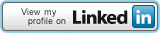## Connect Me## Pages

### C Programs for HSEB 12

ARRAY
/*Program to input values into an array and display them.*/
#include
#include
void main()
{
int arr,i;
clrscr();
for(i=0;i<5;i++) { printf("Enter he value for array[%d] :",i); scanf("%d",&arr[i]); } printf("The array elements are"\n"); for(i=0;i<5;i++) printf("%d\t",arr[i]); printf("\n"); getch(); }

/* Program to add the elements of an array. */
#include
#include
void main()
{
int arr,I,sum=0;
clrscr();
for(i=0;i<10;i++) { printf("Enter the value for arr[%d]:", i); scanf("%d",&arr[i]); sum=sum+arr[i]; } printf("Sum of the elements of array = %d\n",sum); getch(); }

/*Program to count even and odd numbers in a array*/
#include
#include
#define size 10
void main()
{
int arr[size],i,even=0,odd=0;
clrscr();
for(i=0;i

/*Program to find the maximum and minimum number in an array. */
#include
#include
void main()
{
int i,arr={2,5,4,1,8,9,11,6,3,7}
int min,max;
clrscr();
min=max=arr;
for(i=1;i<10;i++) { if(arr[i]max)
max=arr[i];
}
printf("Minimum number in array = %d, Maximum number in array = %d\n",min,max);
getch();
}

/*Program to reverse the elements of an array. */
#include
#include
void main()
{
int i,j,temp,arr={1,2,3,4,5,6,7,8,9,10};
clrscr();
for(i=0,j=9;i

/* Program input and display a matrix */
#include
#include
#define row3
#define col 4
void main()
{
int mat[row][col],i,j;
printf("Enter the elements of matrix[%dx%d] row-wise :\n",row,col);
for(i=0;i

/* Program for addition of two matrices. */
#define row 3
#define col 4
#include
#include
void main()
{
int i,j,mat1[row][col],mat2[row][col],mat3[row][col];
clrscr();
printf("Enter matrix mat1(%dx%d) row-wise : \n",row,col);
for(i=0;i

/* Program for multiplication of two matrices. */
#define row1 3
#define col1 4
#define row2 col1
#define col2 2
#include
#include
void main()
{
int i,j,k,mat1[row1][col1],mat2[row2][col2],mat3[row1][col2];
clrscr();
printf("Enter matrix mat1(%dx%d) row-wise : \n",row1,col1);f
for(i=0;i

STRINGS
/* Program for input and output of strings using scanf() and printf() function. */
#include
#include
#include
void main()
{
char str="Welcome to the world of Strings";
clrscr();
printf("String is : %s\n",str);
printf("Enter new value for string :\n");
gets(str);
printf("New string is : %s\n",str);
getch();
}

/* Program to print characters of a string and address of each characters. */
#include
#include
void main()
{
char str[]="Nepal";
int i;
for(i=0;str[i]!='\0';i++)
{
printf("Character - %c\t", str[i]);
printf("Address = %u\n, str[i]);
}
getch();
}

/*Program to understand the work of strlen() fuction. */
#include
#include
void main()
{
char str;
int length;
clrscr();
printf("Enter the string.\n");
gets(str);
length=strlen(str);
printf("Length of the string is : %d\n", length);
getch();
}

/*Program to understand the work of strcmp() function */
#include
#include
#include
void main()
{
char str1,str2;
printf("Enter the first string :");
gets(str1);
printf("Enter the second string :");
gets(str2);
if((strcmp(str1,str2))==0)
printf("Strings are same.\n");
else
printf("Strings are not same.\n");
getch();
}

/*Program to understand the work of strcpy() function. */
#include
#include
#include
void main()
{
char str1,str2;
clrscr();
printf("Enter the first string :");
gets(str1);
printf("Enter the second string :");
gets(str2);
strcpy(str1,str2);
printf("First string :%s \t\t Second string :%s\n",str1,str2);
strcpy(str1,"Nepal");
strcpy(str2,"Kathmandu");
printf("First string :%s \t\t Second string :%s\n",str1,str2);
getch();
}

/*Program to understand the work of strcpy() function. */
#include
#include
#include
void main()
{
char str1,str2;
clrscr();
printf("Enter the first string :");
gets(str1);
printf("Enter the second string :");
gets(str2);
strcat(str1,str2);
printf("First string :%s \t Second string :%s\n",str1,str2);
strcat(str1,"Computer");
printf("Now the first string is : %s \n",str1);
getch();
}

/*Program to understand the work of strcat() function. */
#include
#include
#include
void main()
{
char str1, str2;
clrscr();
printf("Enter the first string :");
gets(str1);
printf("Enter the second string :");
gets(str2);
printf("First string is : %s \t Second string is : %s\n",str1,str2);
strcat(str1,str2);
printf("Now first string is : %s \n",str1);
getch();
}

/* Program to sort the array of strings. */
#include
#include
#include
#define n 5
#define len 10
void main()
{
char str1, str2;
clrscr();
char arr[n][len]={"white","red","green","yellow","blue"};
char temp;
int i,j;
printf("Before sorting :\n");
for(i=0;i0)
{
strcpy(temp,arr[i]);
strcpy(arr[i],arr[j]);
strcpy(arr[j],temp);
}
printf("After sorting :\n");
for(i=0;i

GENERAL
/*Program to enter length and breadth & print area and perimeter of rectangle.*/
#include
#include
void main()
{
float l,b,a,p;
clrscr();
printf("Enter length and breadth\n");
scanf("%f",&l);
scanf("%f",&b);
a=l*b;
p=2*(l+b);
printf("The area of the rectangle is %f", a);
printf("The perimeter of the rectangle is %f",p);
getch();
}

/* Program to enter your name and address and print them 10 times. */
#include
#include
void main()
{
char n, a;
int i;
clrscr();
scanf("%s",&n);
scanf("%s",&a);
for(i=1;i<=10;i++) { printf("Your name is %s\n", n); printf("Your address is %s\n",a); } getch(); }

/* Program to enter a number and print whether it is EVEN or ODD.*/
#include
#include
void main()
{
int x;
clrscr();
printf("Enter a number\n");
scanf("%d",&x);
if(x%2==0)
printf("The supplied number is EVEN.\n");
else
printf("The supplied number is ODD.\n);
getch();
}

/* Program to enter a number and print whether it is PRIME or COMPOSITE. */
#include
#include
void main()
{
int a,r,i,c=0;
clrscr();
printf("Enter a number\n");
scanf("%d",&a);
for(i=1;i<=a;i++) { r=a%2; if(r==0) c=c+1; } If(c==2) printf("The supplied number %d is prime",a); else printf("The supplied numer %d is composite\n",a); getch(); }

/* Program to enter 3 numbers and print the (greatest)largest number. */
#include
#include
void main()
{
int a,b,c;
clrscr();
If(a>b && a>c)
printf("the greatest number is %d", a);
else if(b>a && b>c)
printf("the greatest number is %d", b);
else
printf("the greatest number is %d", c);
getch();
}

/* Program of sorting using selection sort. */
#define size 10
#include
#include
void main()
{
int arr[size];
int i,j,temp;
printf("Enter elements of the array :\n");
for(i=0;iarr[j])
{
temp=arr[i];
arr[i]=arr[j];
arr[j]=temp;
}
}
printf("Sorted array in Descending Order is : \n");
for(i=0;i

POINTERS
/* Program to print address of variables using address operator. */
#include
#include
void main()
{
int age=24;
float sal=15000.75;
printf("Value of age = %d, Address of variable age = %u\n",age,&age);
printf("Value of sal = %.2f, Address of variable sal = %u\n",sal,&sal);
getch();
}

/* Program to dereference pointer variables. */
#include
#include
void main()
{
int a=100;
float b=4.5;
int *p1=&al;
float *p2=&b;
clrscr();
printf("Value of p1 = Address of a= %u\n", p1);
printf("Value of p2 = Address of b = %u\n",p2);
printf("Address of p1 = %u\n", &p1);
printf("Address of p2 = %u\n",&p2);
printf("Value of a = %d %d %d \n",a,*p1,*(&a));
printf("Value of b = %f %f %f \n", b,*p2, *(&b));
getch();
}

Ą